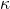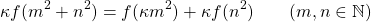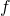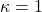# Multiplicative functions satisfying the functional equation κf(m2+n2) = f(κm2) + f(κn2)

Wuttichai Suriyacharoen and Vichian Laohakosol
Notes on Number Theory and Discrete Mathematics
Print ISSN 1310–5132, Online ISSN 2367–8275
Volume 27, 2021, Number 3, Pages 1—11
DOI: 10.7546/nntdm.2021.27.3.1-11

## Details

### Authors and affiliations

Wuttichai SuriyacharoenDepartment of Mathematics and Statistics, Thammasat University
99 Moo 18 Paholyothin Rd, Klong Nueng, Klong Luang, Pathumthani 12121, Thailand

Vichian LaohakosolDepartment of Mathematics, Kasetsart University
50 Ngamwongwan Rd, Chatuchak, Bangkok 10900, Thailand

### Abstract

For a fixed positive integer, the functional equationis solved for multiplicative functions. This complements a 1996 result of Chung  which deals with the case. The method used relies on the sum of two squares theorem in number theory.

### Keywords

• Arithmetic function
• Multiplicative function
• Functional equation
• Sum of squares

• 11A25
• 39B52

### References

1. Bašić, B. (2014). Characterization of arithmetic functions that preserve the sum-of-squares operation. Acta Mathematica Sinica, 4, 689–695.
2. Chung, P. V. (1996). Multiplicative functions satisfying the equation f(m2+n2) = f(m2)+f(n2). Mathematica Slovaca, 46, 165–171.
3. Dubickas, A., & Šarka, P. (2013). On multiplicative functions which are additive on sums of primes. Aequationes Mathematicae, 86, 81–89.
4. Fang, J. H. (2011). A characterization of the identity function with equation
f(p + q + r) = f(p) + f(q) + f(r). Combinatorica, 31, 697–701.
5. Kátai, I.,& Phong, B. M. (2014). The functional equation f(A + B) = g(A) + h(B). Annales Universitatis Scientiarium Budapestinensis de Rolando Eötvös nominatae. Sectio Computatorica, 43, 287–301.
6. Khanh, B. M. M. (2015). On the equation f(n2 + Dm2) = f(n)2 + Df(m)2. Annales Universitatis Scientiarium Budapestinensis de Rolando Eötvös nominatae. Sectio Computatorica, 44, 59–68.
7. Khanh, B. M. M. (2019). A note on a result of B. Bojan. Annales Universitatis Scientiarium Budapestinensis de Rolando Eötvös nominatae. Sectio Computatorica, 49, 285–297.
8. Kim, B., Kim, J. Y., Lee, C. G., & Park, P.-S. (2018). Multiplicative functions additive on generalized pentagonal numbers. Comptes Rendus Mathematique, 356(2), 125–128.
9. McCarthy P. J. (1985). Introduction to Arithmetical Functions. Springer, New York.
10. Niven, I., Zuckerman, H. S., & Montgomery, H. L. (1991). An Introduction to the Theory of Numbers. Wiley, New York.
11. Park, P.-S. (2018). Multiplicative functions commutable with sums of squares. International Journal of Number Theory, 2, 469–478.
12. Park, P.-S. (2018). On k-additive uniqueness of the set of squares for multiplicative functions. Aequationes Mathematicae, 92, 487–495.
13. Spiro, C. (1992). Additive uniqueness sets for arithmetic functions. Journal of Number Theory, 42, 232–246.

## Cite this paper

Suriyacharoen, W., & Laohakosol, V. (2021). Multiplicative functions satisfying the functional equation κf(m2+n2) = f(κm2) + f(κn2). Notes on Number Theory and Discrete Mathematics, 27(3), 1-11, doi: 10.7546/nntdm.2021.27.3.1-11.